### Home > A2C > Chapter Ch11 > Lesson 11.3.3 > Problem11-156

11-156.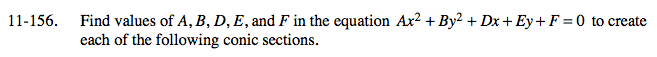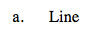Write an equation for a line then convert it into this general form.

y = x
0x2 + 0y2x + y + 0 = 0
A = 0, B = 0, D = −1, E = 1, F = 0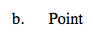See part (a).

What kind of equation defines a point?

x2 + y2 = 0
x2 + y2 + 0x + 0y + 0 = 0
A = 1, B = 1, D = 0, E = 0, F = 0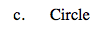See part (a).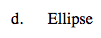See part (a).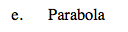See part (a).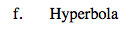See part (a).

y2x2 = 1
x2 + y2 = 0x + 0y −1 = 0
A = −1, B = 1, D = 0, E = 0, F = −1